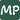# How to calculate the number of days between two dates in javascript?

September 20, 2021

Date manipulation and calculations are core to a huge amount of enterprise business logic. Being a wizard at using dates and turning them into simple values to run your logic on is critical. Knowing how to calculate the number of days between two dates is an essential piece of logic to have in your utility belt. This post will explain clearly how the math works as well as creating a simple utility function that can be used anywhere.

### Understanding the Javascript Date Object

The Javascript date object API is big and provides a wide range of functionality around dates in general. We’re not going to need to dig into it, but it is well worth looking further into for other requirements.

The same goes for the Javascript Math API as it covers a wide range of mathematical operations, but we only need two for this to work.

The key with the below code snippet is that subtracting one date from another will automatically convert the date objects into their base millisecond form to perform the arithmetic.

We can then divide the millisecond subtraction by the number of milliseconds in one day to factor the number down to days.

Using Math.abs to enforce the absolute value of the above calculation will ensure the number of days calculated is a positive number.

``````function daysBetweenTwoDates(firstDate, secondDate) {
/**
* Calculate the number of milliseconds in a day
* 24 hours multipled by 60 minutes an hour
* multiplied by 60 seconds an hour
* multiplied by 1000 milliseconds a second.
*/
const oneDayInMilliseconds = 24 * 60 * 60 * 1000;
return Math.round(Math.abs((firstDate - secondDate) / oneDayInMilliseconds));
}

const firstOfSept = new Date("09/01/2021");
const now = new Date();
const diffDays = daysBetweenTwoDates(now, firstOfSept);
console.log('Number of days is', diffDays);``````Blog by Mick Patterson